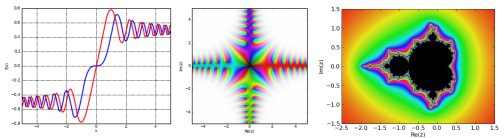#mpmath is a free (BSD licensed) Python library for real and complex floating-point arithmetic with arbitrary precision. It has been developed by Fredrik Johansson since 2007, with help from many contributors.

The following example computes 50 digits of pi by numerically evaluating the Gaussian integral with mpmath. See 100 mpmath one-liners for pi and the documentation links below for many more examples!

```>>> from mpmath import mp
>>> mp.dps = 50
>>> print(mp.quad(lambda x: mp.exp(-x**2), [-mp.inf, mp.inf]) ** 2)
3.1415926535897932384626433832795028841971693993751
```

mpmath works with both Python 2 and Python 3, with no other required dependencies. It can be used as a library, interactively via the Python interpreter, or from within the SymPy or Sage computer algebra systems which include mpmath as standard component. CoCalc lets you use mpmath directly in the browser.

Source code git repository: https://github.com/fredrik-johansson/mpmath

Issue tracker: https://github.com/fredrik-johansson/mpmath/issues

Feedback and questions are welcome on the mpmath mailing list (mpmath@googlegroups.com)

This project was previously hosted on Google Code. See http://code.google.com/p/mpmath/ for the old site.

## Documentation

The documentation provides installation instructions and extensive interactive examples.

Main documentation, current development version (HTML)

## Features

mpmath can be used as an arbitrary-precision substitute for Python's float/complex types and math/cmath modules, but also does much more advanced mathematics. Almost any calculation can be performed just as well at 10-digit or 1000-digit precision, with either real or complex numbers, and in many cases mpmath implements efficient algorithms that scale well for extremely high precision work.

• Numerical integration (regular, double/triple integrals, oscillatory)
• Numerical differentiation and differintegration (arbitrary orders); partial derivatives
• Limits and summation of infinite series (with convergence acceleration)
• Root-finding (1D and multidimensional; secant method, bisection, modified Newton's method, and other algorithms)
• Polynomial evaluation and polynomial root-finding
• Chebyshev approximations, Fourier and Taylor series
• Solving ODEs
• Integer relation detection (constant recognition)
• Linear algebra (linear system solving, LU factorization, matrix inverse, matrix norms, matrix exponentials/logarithms/square roots, eigenvalues, singular values, QR factorization)
• Inverse Laplace transforms

mpmath implements a huge number of special functions, with arbitrary precision and full support for complex numbers:

Elementary functions (sqrt, exp, log, trigonometric, hyperbolic and inverse functions); mathematical constants (pi, e, the golden ratio, Euler's constant gamma, Catalan's, Apery's, Khinchin's and Glaisher's constants); the Lambert W function (all branches); the error function (erf), imaginary and complementary error functions; the inverse error function of a real argument; normal distribution functions; gamma functions (complete and incomplete), factorials, double factorials and binomial coefficients, the log-gamma function; complete and incomplete beta functions; exponential and trigonometric integrals; Fibonacci and Stirling numbers; Barnes G-function, super- and hyperfactorials; polygamma functions; Riemann zeta function (including evaluation with the Riemann-Siegel expansion), Hurwitz zeta function, Riemann-Siegel and related functions; Riemann zeta zeros; Bernoulli numbers (fast numerical and exact computation of large Bernoulli numbers), Bernoulli polynomials, Euler numbers and polynomials; polylogarithms, Clausen functions; Stieltjes constants; Bessel functions; Hankel, Struve, Kelvin, Whittaker, Airy, Coulomb functions; Bessel function zeros; parabolic cylinder functions; the arithmetic-geometric mean; complete and incomplete elliptic integrals (Legendre and Carlsen forms); Jacobi elliptic functions and Jacobi theta functions; Jacobi, Legendre, Chebyshev and other orthogonal polynomials; associated Legendre functions; spherical harmonics; generalized hypergeometric functions; the Meijer G-function; Borel regularized hypergeometric series; bilateral hypergeometric series; 2D hypergeometric series (Appell, Horn, Kampé de Fériet functions); q-factorials and q-hypergeometric series.

mpmath also includes rudimentary support for interval arithmetic (only basic functions are available).

If matplotlib is available, mpmath provides a convenient plotting interface. The pictures at the top of this page were generated by the commands plot([fresnels, fresnelc]), cplot(fresnels), and the mandelbrot.py script. See the function gallery for more images.

mpmath internally uses Python's builtin long integers by default, but automatically switches to GMP/MPIR for much faster high-precision arithmetic if gmpy is installed or if mpmath is imported from within Sage.

## Software using mpmath

The Sage computer algebra system includes mpmath as a standard component, and uses it for numerical evaluation of special functions. SymPy and SympyCore, Python computer algebra systems, use mpmath for numerical evaluation. Other software includes:

• hcpy, an RPN calculator
• pytaylor Taylor models for Python using mpmath and sympy
• NZMATH uses mpmath for auxiliary calculations in its ECPP code

## References to mpmath

As of April 2014, Google Scholar lists over 100 references to mpmath in the scientific literature. A very outdated hand-compiled list can be found here.

If you use mpmath in your research, please cite it! In BibTeX format, the following entry can be used:

```@manual{mpmath,
key     = {mpmath},
author  = {Fredrik Johansson and others},
title   = {mpmath: a {P}ython library for arbitrary-precision floating-point arithmetic (version 0.18)},
note    = {{\tt http://mpmath.org/}},
month   = {December},
year    = {2013},
}
```
This might render as:
• Fredrik Johansson and others. mpmath: a Python library for arbitrary-precision floating-point arithmetic (version 0.18), December 2013. http://mpmath.org/.

Preferably, change the version number to the version you used, and use the date of its release.